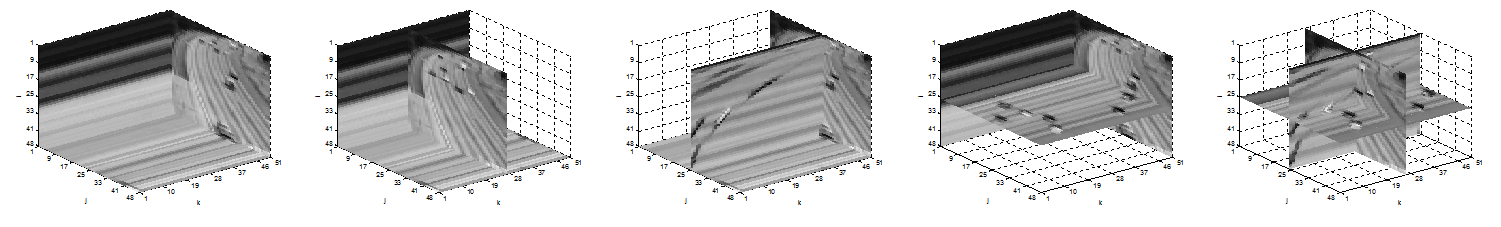# mtt MATLAB Tensor ToolsLast update: 08/09/2014

## MTT

Matlab Tensor Tools is an easy-to-use library to work with tensors.``````See also:

Presentation about Matrix and Tensor Tools for Computer Vision
http://www.slideshare.net/andrewssobral/matrix-and-tensor-tools-for-computer-vision

LRSLibrary: Low-Rank and Sparse Tools for Background Modeling and Subtraction in Videos
https://github.com/andrewssobral/lrslibrary

IMTSL: Incremental and Multi-feature Tensor Subspace Learning
https://github.com/andrewssobral/imtsl
``````

## Citation

If you use this code for your publications, please cite it as:

``````@misc{asobral2014,
author       = "Sobral, Andrews",
title        = "Matlab Tensor Tools",
year         = "2014",
url          = "https://github.com/andrewssobral/mtt/"
}
``````

## Demos

``````tensor_demo_operations.m - Basic operations
tensor_demo_hosvd_ihosvd.m - High-order singular value decomposition (Tucker decomposition)
tensor_demo_parafac_als.m - CP decomposition via ALS (Alternating Least-Squares)
tensor_demo_tucker_als.m - Tucker decomposition via ALS (Alternating Least-Squares)
tensor_demo_tsvd.m - t-SVD and inverse t-svd
tensor_demo_ntf.m - Non-Negative Tensor Factorization
tensor_demo_subtensors_ntf_hals.m - Low-rank approximation based Non-Negative Tensor(CP) factorization
tensor_demo_inclearn.m - Incremental tensor learning
``````

## Example of tensor operations

```A = reshape(1:12,[2,2,3]);
B = reshape(1:12,[2,2,3]);

%% Basic operations
[A1,A2,A3] = tensor_matricization(A);

M22 = reshape(1:4,[2,2]);
M33 = reshape(1:9,[3,3]);
B1 = tensor_nmodeproduct(A,M22,1);
B2 = tensor_nmodeproduct(A,M22,2);
B3 = tensor_nmodeproduct(A,M33,3);

Au = tensor_unfold(A);
A_hat = tensor_fold(Au,size(A));

[A1_] = tensor_slices_frontal(A);
[A2_] = tensor_slices_lateral(A);
[A3_] = tensor_slices_horizontal(A);

[At1_] = tensor_fibers_column(A);
[At2_] = tensor_fibers_row(A);
[At3_] = tensor_fibers_tube(A);

Bt = tensor_transpose(B);
[C] = tensor_product(A,B);

%% HoSVD and iHoSVD decomposition
T = tensor(A);
[core,U] = tensor_hosvd(T);
[T_hat] = tensor_ihosvd(core,U);

%% t-SVD decomposition
[U,S,V] = tensor_t_svd(A);
[C] = tensor_product(U,S);
[A_hat] = tensor_product(C,tensor_transpose(V));

%% Tucker ALS decomposition
r = 10;
T_hat = tucker_als(T,[r r r]);

%% PARAFAC/CP ALS decomposition
T_hat = cp_als(T, r);```